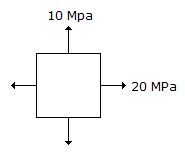# Civil Engineering - UPSC Civil Service Exam Questions

6.

The velocity distribution for flow over a plate is given by u = 0.5 y - y2 where u is the velocity in m/s at a distance y metre above the plate. If the dynamic viscosity of the fluid is 0.9 N/m2, then what is the shear stress at 0.20 m from the boundary?

 A. 0.9 N/m2 B. 1.8 N/m2 C. 2.25 N/m2 D. 0.09 N/m2

Explanation:

No answer description available for this question. Let us discuss.

7.

Consider the following :
1. Initial consolidation.
2. Primary consolidation.
3. Secondary consolidation.
4. Final consolidation.
The three stages which would be relevant to consolidation of a soil deposit includes

 A. 1, 2 and 3 B. 2, 3 and 4 C. 1, 3 and 4 D. 1, 2 and 4

Explanation:

No answer description available for this question. Let us discuss.

8.

Consider the following statements :
1. Mastic asphalt is a mixture of hard grade bitumen or blown bitumen, minerals filter and fine aggregates.
2. % of binder content in the mastic asphalt is 17-20 percent by weight of the aggregates. Which of the statements given above is/are correct ?

 A. 1 only B. 2 only C. Both 1 and 2 D. Neither 1 nor 2

Explanation:

No answer description available for this question. Let us discuss.

9.

An element is subjected to stress as given in figure. For this state of stress, what is the maximum shear stress ?A. 2.5 MPa B. 5 MPa C. 10 MPa D. 15 MPa

Explanation:

No answer description available for this question. Let us discuss.

10.

What is the maximum possible value of Poisson's ratio for a non-dilatant material ?

 A. 0.67 B. 0.5 C. 0.33 D. 0.25